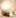Factor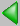- - - - You are here: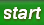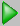FFactor - - - -Topic Index A B C D E F G H I J K L M N O P Q R S T U V W X Y Z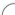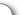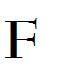# Factor

(1)

## What are the factors?

Factor, Key Factor.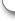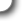The root word: Factor     The key word: Factors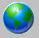Topics from our world ...Key Factor This is a key factor.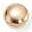What do you think about: Factor?
Email: Web @ RRooks.us Subject: F.Factor
Please submit your ideas, suggestions, and comments: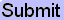- - - - You are here:FFactor - - - -Last Revision: July 06, 2010 D=2, W=9, F=0, I=1, E=0, V=0, C=0, S=3 F Topic: 3 of 54 = Factor